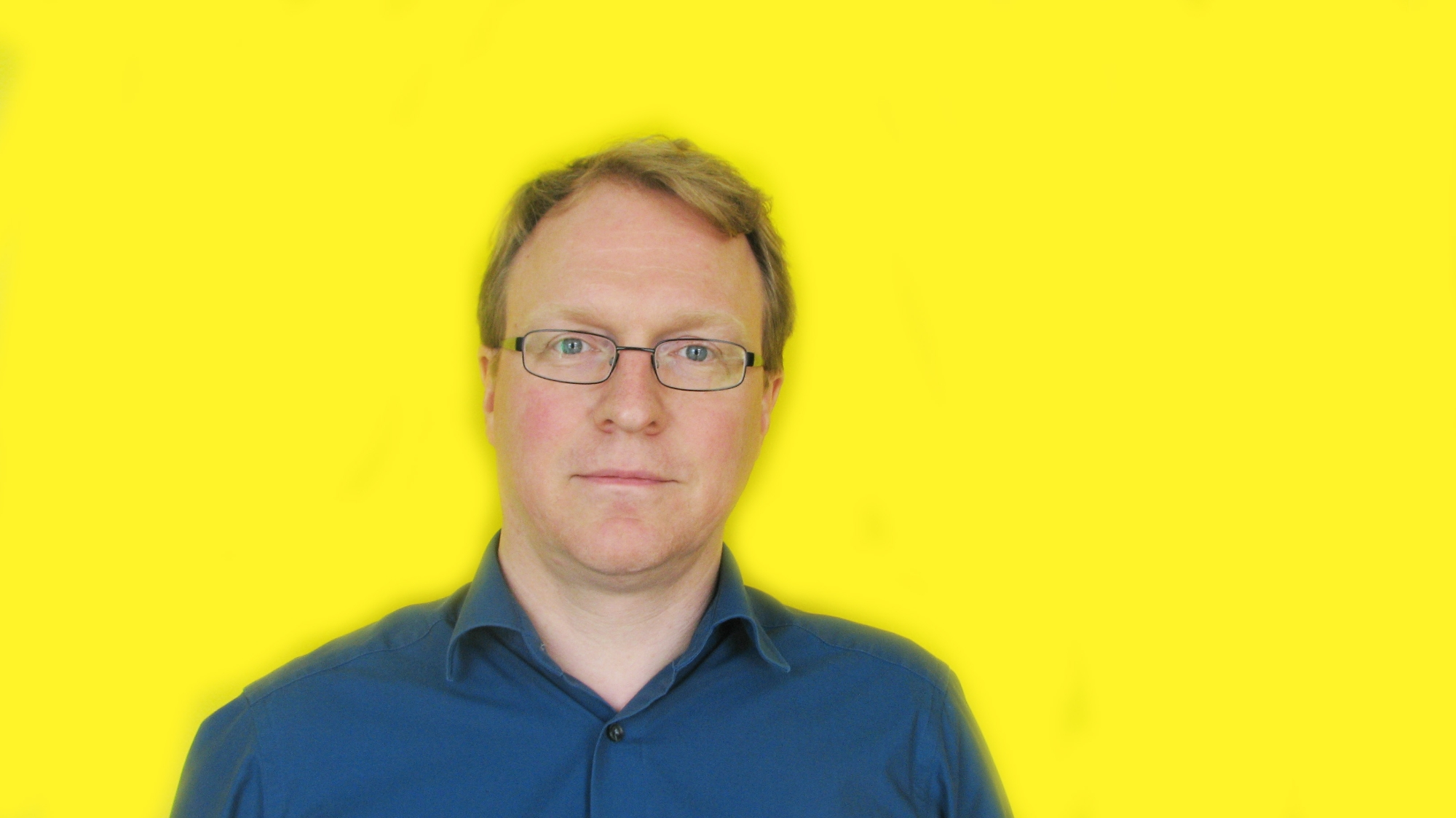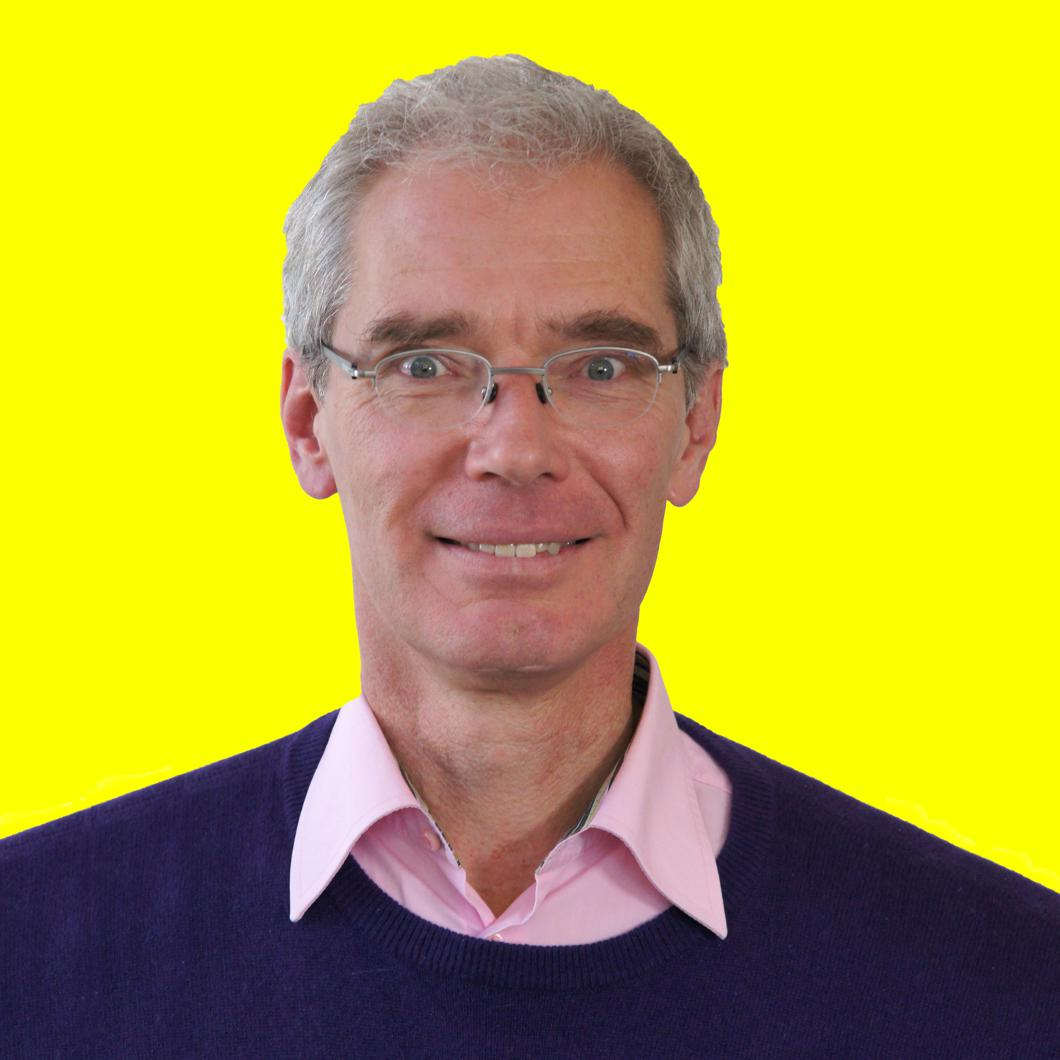# THz Spectroscopy

A short introduction to the experimental THz spectroscopy method

### Specifications

 Frequency range: 1 - 40 cm-1 30 GHz - 1.2 THz Temperature interval: 2 - 300 K Magnetic field: up to 8 Tesla Measurable spectra: complex refractive index complex dielectric permittivity complex conductivity surface resistance/reactance transmission/reflection coefficient

The measurements can be performed in the frequency range 1 - 40 cm-1 (i.e., 30 - 1200 GHz, which corresponds to an energy range 0.1 - 5 meV or to a wavelengths 250 µm - 10 mm) at temperatures T = 2 - 300 K (custom-built cryostat), in an external magnetic fields up to 8 T (Oxford Spectromag 4000) and at elevated temperatures up to 1000 K (home-made ovens). The measurements are fully computer-controlled and rapidly done in a real-time scale.

As sources of the electromagnetic radiation the spectrometer utilizes powerful backward-wave oscillators (BWOs). BWOs produce monochromatic, polarized radiation which can be continuously tuned in frequency over a wide range. The whole range from 1 to 40 cm-1 is covered by 12 different BWOs as shown in Fig.1 below.

The frequency of the BWO can be tuned by scanning the high voltage and can be precisely measured with a quasioptical tunable Fabry-Perot resonators. We use power supplies providing tunable high voltage up to 5.5 kV, with noise, ripples and short-term deviations not exceeding 10 - 15 mV leading to the output frequency stability Df / f ~ 10-5 - 10-6.

The lock-in registration system includes the radiation detector (Golay cell or He-cooled bolometer) and sampling­ storage­ reset electronics. The dynamic range of the registration system is about 104 which together with the dynamic range of the quasioptical attenuator unit provides a full dynamic range of the spectrometer of 106 - 107.

The idea of the submm dielectric measurements is based on measurement of the spectra of two quantities characterizing the interaction of the electromagnetic field with the plane-parallel sample: the power transmission coefficient and the phase shift of the radiation upon transmission through the sample. This allows one to determine the dielectric properties of the sample directly, without using the Kramers-Kronig procedure. The mathematical relationships connecting the transmission coefficient Tr and the phase with the dielectric parameters of the sample (refracive index, dielectric permittivity, conductivity, etc.) are well known and can be found in any textbook on optics or electrodynamics . After the Transmission and Phase spectra are measured, the dielectric spectra of complex refractive index n + i k of the sample are calculated directly by solving the set of two equations with two unknowns (n and k), at each frequency in the chosen range.

The measurement scheme is a standard optical setup: The radiation generated by the BWO is collimated into a parallel beam by a Teflon or polyethylene lens; it propagates through free space and is finally measured by the detector. Plane wire grids made out of thin tungsten wires spaced at a distance much smaller than the radiation wavelength are used as polarizers, analyzers, semi-transparent mirrors and beam-splitters. The radiation is focussed on the sample by a Teflon or polyethylene lenses. For measurement of the transmitted power only the "red part" of the spectrometer (see Fig. 2) is employed and the signal on the detector is recorded versus frequency two times - without and with the sample. The absolute transmission coefficient is determined as a ratio of the two correspondent data arrays. To measure the phase shift all elements of the spectrometer are used forming a Mach-Zehnder two-beam polarization interferometer. One of the mirrors of the interferometer can be moved; its position is controlled by an electronic feedback system in such a way that during the frequency scanning the interferometer is always kept in a balanced state (equal optical paths in its both arms). The quantity measured in this phase-shift mode is the position of this movable mirror. Again, the frequency is scanned twice - without and with the sample - and the phase-shift spectrum is determined on the basis of the correspondent difference of the two positions of the mirror.

Fig. 4 shows an example of how dielectric parameters (real and imaginary parts of dielectric permittivity) can be determined by measurement of the transmissivity and the phase shift of a plane-parallel sample.

By this method practically any material can be measured as long as the power of the submm radiation which penetrates the sample (transmission coefficient) is at the level of 10-4 (with the Golay cell as the detector) or 10-6 - 10-7 (using a He-bolometer). Assuming that the bulk sample can be polished down to the thickness of about 10 microns (see for example [3,4]) then dielectric permittivity up to 1000 (conductivity up to 300 Ohm-1cm-1) are accessible for measurements by the submm spectrometer in the transmission geometry with the average accuracy of 5 % for the dielectric permittivity and 10 % for the conductivity.

The submm measurements can be done simpler and more rapidly if the samples are transparent for the submm radiation to an extent that interference fringes can be observed in the transmission coefficient spectra in the form of periodic maxima. These maxima appear due to multiple reflections of the radiation inside the sample, which in this case serves as a multibeam dielectric-filled Fabry-Perot resonator. An example of such spectrum is shown in the Fig. 5 and another example - in Fig. 6 where a deep minimum is superimposed on interference pattern corresponding to a magnetic mode.

It can be easily shown  that the frequency difference between the maxima is determined by the refractive index n and the thickness d of the sample via m l  = 2 n d where m is the order of the interference and l is the wavelength of the radiation. The amplitudes of the interference maxima is given by the extinction coefficient k. This allows the determination of both n and k by a least-square-fit of the data using the expression which describes the transmissivity of a plane-parallel layer to the measured spectrum. No extra phase measurement is required, however it may improve the accuracy of the fit.

Measurement of transmission and phase shift allows also to determine the spectra of conductivity and permittivity of thin films on transparent substrates (two layer system). Since films often can be made very thin, practically any material can be measured, even metallic or superconducting films. In this case the dielectric properties of the substrate are measured beforehand. In a second step the transmissivity and the phase shift of the film on the substrate are measured in a usual way described above and then the film properties can be extracted from these spectra by using the expressions for complex transmission coefficient of the two-layered system (film on the substrate). An example of such measurement is shown in the Fig. 7.

When needed, instead of the transmissivity, direct reflectivity measurements can be performed utilizing the spectrometer. The corresponding layout is presented in the Fig. 8.

Here the radiation is focused on the sample by a metallic parabolic mirror. Using a semi-transparent wire-grid beamsplitter the intensity is recorded versus frequency by the detector after been reflected off the plane sample and off the reference mirror. The reflection coefficient is obtained by division of the two data arrays. For these measurements a special reflection unit is employed. In the Fig. 9 below we show a typical example of direct measurement of reflection coefficient at mm-submm wavelengths performed using several BWOs.

References:

1. G.Kozlov, A.Volkov. Coherent Source Submillimeter Wave Spectroscopy. in: Millimeter and Submillimeter Spectroscopy of Solids, Ed. by G.Grüner (Topics in Applied Physics, vol.74, Springer Verlag Berlin Heidelberg 1998)
2. M.Born, E.Wolf. Principles of Optics, Pergamon Press Oxford 1986;
Jackson, Classical Electrodynamics, Wiley and Sons
3. B. Gorshunov et al., Solid State Commun. 60 681 (1986)
4. B. Gorshunov, N. Sluchanko, A. Volkov, M. Dressel, G. Knebel, A. Loidl, and S. Kunii, Phys. Rev. B 59 1808 (1999)
5. A.V. Pronin, M. Dressel, A. Pimenov, A. Loidl, I.V. Roshchin, and L.H. Greene, Phys.Rev. B 57, 14416

## Figures to detailed description

### ContactDr.

### Marc Scheffler

#### Head of the Microwave LabProf. Dr. rer. nat.